## ML Aggarwal Class 8 Solutions for ICSE Maths Chapter 3 Squares and Square Roots Ex 3.3

Question 1.
By repeated subtraction of odd numbers starting from 1, find whether the following numbers are perfect squares or not? If the number is a perfect square then find its square root:
(i) 121
(ii) 55
(iii) 36
(iv) 90
Solution:
(i) Square root of 121
121 – 1 = 120
120 – 3 = 117
117 – 5 = 112
112 – 7 = 105
105 – 9 = 96
96 – 11 = 85
85 – 13 = 72
72 – 15 = 57
57 – 17 = 40
40 – 19 = 21
21 – 21 =0
∴ Square root of 121 is 11
∴ 121 is a perfect square.

(ii) Square root of 55
55 – 1 = 54
54 – 3 = 51
51 – 5 = 46
46 – 7 = 39
39 – 9 = 30
30 – 11 = 19
19 – 13 = 6
6 – 15 = …… not possible
∴ 55 is not a perfect square.

(iii) Square root of 36
36 – 1 = 35
35 – 3 = 32
32 – 5 = 27
27 – 7 = 20
20 – 9 = 11
11 – 11=0
∴ 36 is a perfect square and its square root is 6.

(iv) Square root of 90
90 – 1 = 89
89 – 3 = 86
86 – 5 = 81
81 – 7 = 74
74 – 9 = 65
65 – 11 = 54
54 – 13 = 41
41 – 15 = 26
26 – 17 = 9
9 – 19 = ….. not possible.
∴ 90 is not a perfect square.

Question 2.
Find the square roots of the following numbers by prime factorisation method:
(i) 784
(ii) 441
(iii) 1849
(iv) 4356
(v) 6241
(vi) 8836
(vii) 8281
(viii) 9025
Solution:
(i) The square root of 784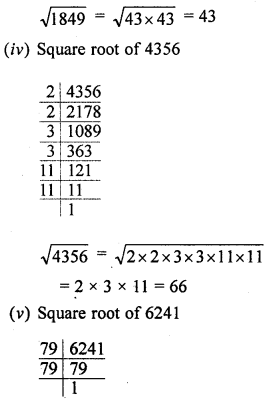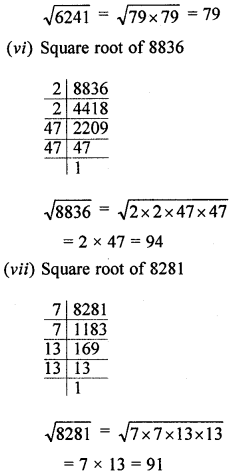Question 3.
Find the square roots of the following numbers by prime factorisation method: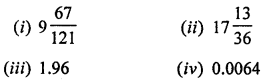Solution: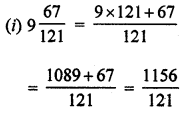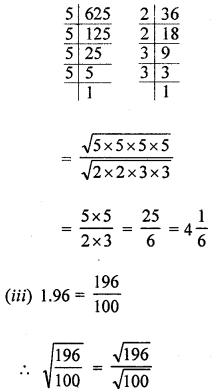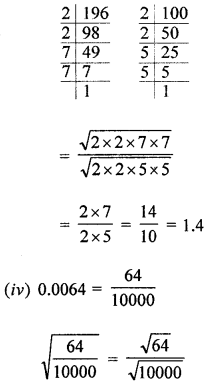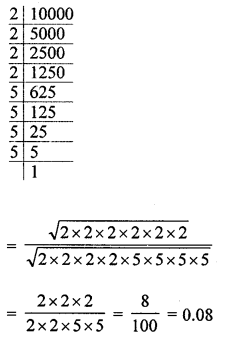Question 4.
For each of the following numbers, find the smallest natural number by which it should be multiplied so as to get a perfect square. Also, find the square root of the square number so obtained:
(i) 588
(ii) 720
(iii) 2178
(iv) 3042
(v) 6300
Solution:
(i) 588 = 2 × 2 × 3 × 7 × 7Pairing the same kind of factors,
we see that one factor 3 is left unpaired.
∴ In order to make it a pair, it must be multiplied by 3.
∴ Required least number = 3
and the square root of 588 × 3 = 1764
= 2 × 3 × 7 = 42

(ii) 720 = 2 × 2 × 2 × 2 × 3 × 3 × 5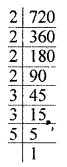Pairing the same kind of factors, we see that one factor 5 is left unpaired.
∴ In order to make it a pair, it must be multiplied by 5.
∴ Required least number = 5
and square root of 720 × 5 = 3600
= 2 × 2 × 3 × 5 = 60

(iii) 2178 = 2 × 3 × 3 × 11 × 11Pairing the same kind of factors, one factor
2 is left unpaird.
∴ The required least number to be multiplied = 2
and the square root of 2178 × 2 = 4356
= 2 × 3 × 11 = 66

(iv) 3042 = 2 × 3 × 3 × 13 × 13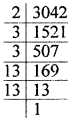Pairing the same kind of factors one factor 2 is left unpaired.
∴ The required least number to be multiplied = 2.
The square root of 3042 × 2 = 6084
= 2 × 3 × 13 = 78

(v) 6300 = 2 × 2 × 3 × 3 × 5 × 5 × 7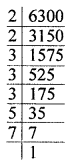Pairing the same kind of factors one factor 7 is left unpaired.
∴ The required least number to be multiplied = 7
The square root of 6300 × 7 = 44100
= 2 × 3 × 5 × 7 = 210

Question 5.
For each of the following numbers, find the smallest natural number by which it should be divided so that this quotient is a perfect square. Also, find the square root of the square number so obtained:
(i) 1872
(ii) 2592
(iii) 3380
(iv) 16224
(v) 61347
Solution:
(i) 1872 = 2 × 2 × 2 × 2 × 3 × 3 × 13Pairing the same kind of factors, one factor 13 is left unpaired.
∴ Required least number = 13.
So, 1872 be divided by 13, the resultant number will be a perfect square.
∴ Resultant number = 1872 ÷ 13 = 144
and square root = 2 × 2 × 3 = 12

(ii) 2592 = 2 × 2 × 2 × 2 × 2 × 3 × 3 × 3 × 3Pairing the same kind of factors, one factor 2 is left unpaired.
∴ Required least number = 2
and the resultant number = 2592 ÷ 2= 1296
and square root = 2 × 2 × 3 × 3 = 36

(iii) 3380 = 2 × 2 × 5 × 13 × 13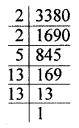Pairing the same kind of factors, one factor 5 is left unpaired.
∴ The required least number = 5
and the resultant number = 3380 ÷ 5 = 676
and the square root = 2 × 13 = 26

(iv) 16224 = 2 × 2 × 2 × 2 × 2 × 3 × 13 × 13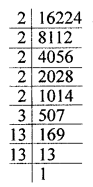Pairing the same kind of factors, two factors 2 and 3 are left unpaired.
So, the required least number = 2 × 3 = 6
and the resultant number = 16224 ÷ 6 = 2704
and square root = 2 × 2 × 13 = 52

(v) 61347 = 3 × 11 × 11 × 13 × 13Pairing the same kind of factors, one factor 3 is left unpaired.
So, the required least number is 3.
The resultant number = 61347 ÷ 3 = 20449
and the square root = 11 × 13 = 143

Question 6.
Find the smallest square number that is divisible by each of the following numbers:
(i) 3, 6, 10, 15
(ii) 6, 9, 27, 36
(iii) 4, 7, 8, 16
Solution:
(i) 3, 6, 10, 15
The number which is divisible by
3, 6, 10, 15 = The LCM of 3, 6, 10, 15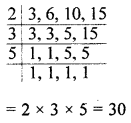and smallest square number which is divisible by 30 = 30 × 30 = 900

(ii) 6, 9, 27, 36
The number which is divisible by 6, 9, 27, 36 is their LCM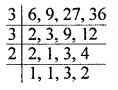= 3 × 3 × 2 × 2 × 3 = 108
and the smallest square
= 108 × 3 = 324

(iii) 4, 7, 8, 16
The number which is divisible by 4, 7, 8, 16 = LCM of their numbers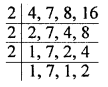= 2 × 2 × 2 × 2 × 7 = 112
The smallest square =112 × 7 = 784

Question 7.
4225 plants are to be planted in a garden in such a way that each row contains as many plants as the number of rows. Find the number of rows and the number of plants in each row.
Solution:
Total number of plants = 4225
∵ The number of rows = Number of the plant in each row.
Number of rows = Square root of 4225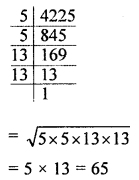Number of rows = 65
and number of plants in each row = 65

Question 8.
The area of a rectangle is 1936 sq. m. If the length of the rectangle is 4 times its breadth, find the dimensions of the rectangle.
Solution:
Area of a rectangle = 1936 sq. m
Then length = 4x m
∴ 4x2 = 1936 ⇒ 4 × 2 = 1936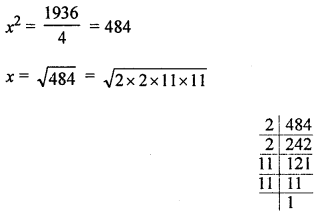= 2 × 11 = 22
Length = 4x = 4 × 22 = 88 m
and breadth = x = 22 m

Question 9.
In a school a P.T. teacher wants to arrange 2000 students in the form of rows and columns for P.T. display. If the number of rows is equal to number of columns and 64 students could not be accommodated in this arrangement. Find the number of rows.
Solution:
Total number of students in a school = 2000
The P.T. teacher wants to arrange them in such a way,
the numbers of rows = Number of students in each row,
∴ By doing this, 64 students are lefts
∴ Required number of students = 2000 – 64 = 1936
and number of rows = $$\sqrt{1936}$$Question 10.
In a school, the students of class VIII collected ₹2304 for a picnic. Each student contributed as mdny rupees as the number of students in the class. Find the number of students in the class.
Solution:
In a school; students of class VIII collected for contribution = ₹2304
Number of students = Number of rupees contribute each student
∴ Number of students = $$\sqrt{2304}$$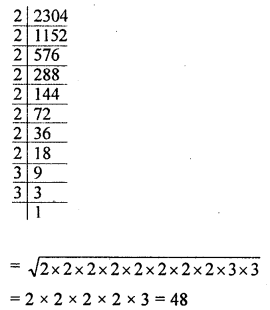Hence number of students in class VIII = 4811.

Question 11.
The product of two numbers is 7260. If one number is 15 times the other number, find the numbers.
Solution:
Product of two numbers = 7260
Let one number = x
Then second = 15x
∴ 15x × x = 7260 ⇒ 15x2 = 7260∴ One number = 22
and second number = 22 × 15 = 330

Question 12.
Find three positive numbers in the ratio 2 : 3 : 5, the sum of whose squares is 950.
Solution:
Ratio in three numbers = 2 : 3 : 5
Sum of their square = 950
Let first number = 2x
Second number = 3x
and third number = 5x
∴ (2x)2 + (3x)2 + (5x)2 = 950
⇒ 4x2 + 9x2 + 25x2 = 950
⇒ 38x2 = 950 ⇒ x2 = $$\frac{950}{38}$$ = 25
x = $$\sqrt{25}$$ = 5
First number = 2 × 5 = 10
Second number = 3 × 5 = 15
Third number = 5 × 5 = 25

Question 13.
The perimeter of two squares are 60 metres and 144 metres respectively. Find the perimeter of another square equal in area to the sum of the first two squares.
Solution:
Perimeter of first square = 60 m
Side = $$\frac{60}{4}$$ = 15 m
and second square = 144
and side = $$\frac{144}{4}$$ = 36 m
∴ Sum of perimeters of two squares
= 60 + 144 = 204 m
and sum of areas of these two squares
= 152 m2 + 362 m2
= 225 m+ 1296 m2 = 1521 m2
∴ Area of third square = 1521 m2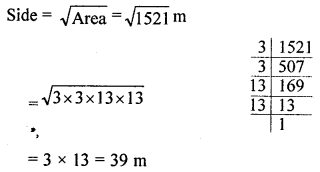∴ Perimeter = 3 × Side
= 4 × 39 = 156 m